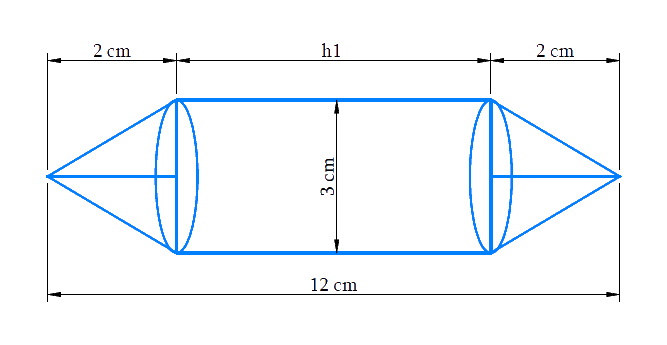# Ex.13.2 Q2 Surface Areas and Volumes Solution - NCERT Maths Class 10

Go back to  'Ex.13.2'

## Question

Rachel, an engineering student, was asked to make a model shaped like a Cylinder with two cones attached at its two ends by using a thin aluminum Sheet. The diameter of the model is $$3\,\rm{cm}$$ and its length is $$12\,\rm{cm}$$. If each cone has a height of $$2\,\rm{cm}$$, find the volume of air contained in the model that Rachel made. (Assume the outer and inner dimensions of the model to be nearly the same.)

## Text Solution

What is known?

Rachel made a model of aluminum sheet shaped like a cylinder with $$2$$ cones attached to its $$2$$ ends.

Diameter of the model $$=3\,\rm{cm}$$

Length of the model $$= 12\,\rm{cm}$$

Height of each cone $$=2\,\rm{cm}$$

The internal and external dimensions of model are same.

What is unknown?

Volume of air contained in the model made by Rachel.

Reasoning:

Draw a figure according to given data to visualize the model.From the figure it’s clear that the volume of the model includes volume of the cylindrical part and both the identical conical parts.

Volume of the model $$=$$ Volume of the $$2$$ conical parts $$+$$ volume of the cylindrical part

Hence, we work on the assumption that the outer and inner dimensions of the model are nearly the same.
Therefore, Volume of air in the model $$=$$ volume of the model based on outer dimensions

Since, length of the model includes height of the cylindrical part and height of both the identical conical parts and also diameter of the cylindrical part and conical parts are same as the diameter of the model.

Length of the model $$=$$ Height of the cylindrical part $$+ {\rm{ }}2 \times$$  Height of the conical part

We will find the volume of the model by using formulae;

Volume of the cylinder  $$= \pi {r^2}{h_1}$$

where $$r$$ and $$h1$$ are the radius and height of the cylinder respectively.

Volume of the cone \begin{align} = \frac{1}{3}\pi {r^2}{h_2}\end{align}

where $$r$$ and $$h2$$ are the radius and height of the cone respectively.

Steps:

Height of each conical part,  $${h_2} = 2cm$$

Height of cylindrical part $$=$$ Length of the model $$- {\rm{ }}2 \times$$ Height of the conical part

\begin{align}{h_1} &= 12cm - 2 \times 2cm\\&= 8cm\end{align}

Diameter of the model,  $$d = 3cm$$

Radius of cylindrical part $$=$$ radius of conical part \begin{align} = r = \frac{{3cm}}{2} = 1.5cm\end{align}

Volume of the model $$=$$ $$2 \times$$ Volume of the conical part $$+$$ volume of the cylindrical part

\begin{align}&= 2 \times \frac{1}{3}\pi {r^2}{h_2} + \pi {r^2}{h_1}\\&= \pi {r^2}\left( {\frac{2}{3}{h_2} + {h_1}} \right)\\&= \frac{{22}}{7} \times 1.5cm \times 1.5cm \times \left( {\frac{2}{3} \times 2cm + 8cm} \right)\\&= \frac{{22}}{7} \times 1.5cm \times 1.5cm \times \frac{{28}}{3}cm\\&= 66c{m^3}\end{align}

Volume of air in the model is $$66\,\rm{cm}^3.$$

Learn from the best math teachers and top your exams

• Live one on one classroom and doubt clearing
• Practice worksheets in and after class for conceptual clarity
• Personalized curriculum to keep up with school